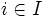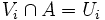# Orthocompactness is weakly hereditary

This article gives the statement, and possibly proof, of a topological space property (i.e., orthocompact space) satisfying a topological space metaproperty (i.e., weakly hereditary property of topological spaces)
View all topological space metaproperty satisfactions | View all topological space metaproperty dissatisfactions
Get more facts about orthocompact space |Get facts that use property satisfaction of orthocompact space | Get facts that use property satisfaction of orthocompact space|Get more facts about weakly hereditary property of topological spaces

## Statement

### Property-theoretic statement

The property of being an orthocompact space is a weakly hereditary property of topological spaces.

### Verbal statement

Any closed subset of a paracompact space is a paracompact space with the subspace topology.

## Proof

### Proof in terms of open covers

Given:$X$ a orthocompact space,$A$ a closed subset of$X$ (with the subspace topology)

To prove: Consider an open cover of$A$ by open sets$U_i$ with$i \in I$, an indexing set. The$U_i$ have an open refinement with the property that at any point, the intersection of all members of the refinement is open.

Proof:

1. By the definition of subspace topology, we can find open sets$V_i$ of$X$ such that$V_i \cap A = U_i$, thus the union of the$V_i$s contains$A$.
2. Since$A$ is closed, we can throw in the open set$X \setminus A$, and get an open cover of the whole space$X$.
3. Since the whole space is orthocompact, this open cover has a refinement with the property that the intersection of all open subsets containing a point is open.
4. By throwing out any member of this cover that is contained in$X \setminus A$, we get an open refinement of the$V_i$ with the property that the intersection of all open subsets containing any point is open.
5. Consider the open cover of$A$ obtained by intersecting each member of this refinement with$A$. This is an open cover of$A$. Moreover, it is a refinement of the$U_i$ (the point here is that the intersection with$A$ of a subset contained in$V_i$ is contained in$U_i$). Finally, it continues to be true that the intersection of the open subsets containing any point is still open. This completes the proof.